Uniform-risk-targeted seismic design for collapse safety
of building structures

SHI Wei, LU XinZheng, YE LiePing

## Department of Civil Engineering, the Key Laboratory of Civil Engineering Safety and Durability of Education Ministry, Tsinghua University, Beijing 100084, PR China

Science China Technological Sciences,2012, 55(6): 1481-1488.Abstract: Seismic design should quantitatively evaluate and control the risk of earthquake-induced collapse that a building structure may experience during its design service life. This requires taking into consideration both the collapse resistant capacity of the building and the earthquake ground motion demand. The fundamental concept of uniform-risk-targeted seismic design and its relevant assessment process are presented in this paper. The risks of earthquake-induced collapse for buildings located in three seismic regions with the same prescribed seismic fortification intensity but different actual seismic hazards are analyzed to illustrate the engineering significance of uniform-risk-targeted seismic design. The results show that with China��s current seismic design method, the risk of earthquake-induced collapse of buildings varies greatly from site to site. Additional research is needed to further develop and implement the uniform-risk-targeted seismic design approach proposed in this paper.

Keyword: building structure, collapse safety, collapse resistant capacity, seismic hazard, uniform-risk-targeted seismic designCitation: Shi W, Lu X Z, Ye L P. Uniform-risk-targeted seismic design for collapse safety of building structures. Sci China Tech Sci, 2012, 55(6): 1481-1488.1. Introduction Investigations on earthquake damage show that the collapse of building structures is the primary source of casualties and property losses during and after a severe earthquake. Therefore, ensuring the collapse safety of building structures is a critical objective of earthquake engineering [1-2]. In recent years, collapse fragility analysis (CFA) [3-5] based on incremental dynamic analysis (IDA)  has been widely used due to its excellent capacity in quantitatively evaluating resistance to earthquake-induced collapse. Through combining the results of a collapse fragility analysis with information on the seismic hazard of the site where the building is located, the risk of earthquake-induced collapse of the investigated building during its entire design service life can be quantitatively evaluated and controlled. This is the fundamental concept of uniform-risk-targeted seismic design [7-8]. The risk of earthquake-induced collapse is measured by the total probability that the building may collapse due to earthquakes in Y years (e.g., 50 years). The objective of uniform-risk-targeted seismic design is to ensure that all buildings throughout a nation should have a uniform acceptable level of risk of earthquake-induced collapse, although they may be located in different seismic regions with different seismic hazards. Seismic design of building structures in China is performed according to the seismic zonation map of China. As a simplified expression of the seismic hazard, the seismic zonation map identifies the seismic fortification intensity for each seismic region as well as the corresponding ground motion values for design purposes. The current Chinese seismic zonation map is defined on the basis of earthquakes with a 10% probability of being exceeded in 50 years (referred to as the fortification-level earthquake) [9-10], while the collapse-prevention-level ground motion values are extrapolated from the fortification-level values [9-10]. Although current Chinese seismic design based on the fortification-level earthquake already takes into account the safety requirements to prevent the earthquake-induced collapse, it sometimes fails to comprehensively account for the variation in seismic hazard due to the complex nature of earthquake mechanisms. Specifically, it is likely that in some regions, the extrapolated collapse-prevention-level ground motion values cannot satisfy the exceedance probability of 2% in 50 years as required in the Chinese Code for Seismic Design of Buildings  . Consequently, buildings in some regions that are designed in accordance with the code  may have a high risk of earthquake-induced collapse. Furthermore, even if the ground motion values for collapse-prevention-level earthquakes were accurately defined using a seismic hazard analysis, the collapse risk of building structures still would not be geographically uniform throughout the nation because the hazard of a mega-earthquake (i.e., an earthquake more intensive than the collapse-prevention-level earthquake)  is different for different regions. Before 1997, the seismic zonation of the United States was mapped based on the earthquakes with an exceedance probability of 10% in 50 years. Since 1997, the maximum considered earthquake (MCE), corresponding to an exceedance probability of 2% in 50 years, has been defined as the baseline for the seismic zonation of the United States. Recently, some adjustments have been applied to MCE ground motions to achieve a uniform collapse risk of 1% collapse probability in 50 years  . The development of the current seismic zonation map of the United States provides a good model for future seismic zonation mapping of China. This paper first presents the basic concept of IDA-based collapse fragility analysis. Second, three cities with the same seismic fortification intensity but different seismic hazards are selected for analysis. The collapse risks of a given reinforced concrete (RC) frame structure located in each of the three cities are predicted and compared. The result shows that though the three cities have the same seismic fortification intensity, the collapse risks of the same building in the three cities are quite different. Additional research is required to develop the uniform-risk-targeted seismic design approach proposed in this paper.

2.       Collapse fragility analysis based on IDA

2.1     Basic process

Collapse fragility analysis [3-5] based on IDA  involves the following four steps: (a) subjecting a structural model to a set of Ntotal earthquake ground motion records, (b) increasingly scaling each ground motion to multiple levels of intensity, (c) implementing nonlinear time-history analyses, and (d) obtaining the collapse probabilities versus intensity levels for further statistical analysis. At a certain intensity level, the number of ground motions that will result in structural collapse is referred to as Ncollapse. The collapse probability at this intensity level is estimated as Pcollapse=Ncollapse/Ntotal. By step-by-step scaling of the intensity level, the complete sequence of the building��s structural behavior can be investigated, from elasticity to yielding to collapse. Meanwhile, the serial collapse probabilities versus incremental intensity levels are obtained (see the data points shown in Figure 1(a)). By assuming a rational probability distribution (e.g., a lognormal distribution [4-5]), the cumulative distribution function of the selected intensity measure (IM) corresponding to structural collapse, referred to as the collapse fragility curve as shown in Figure 1(a), is obtained by statistical methods. The collapse fragility curve is a rational representation of the structure��s collapse resistant capacity, and its reliability depends on the selection and the total number of the ground motion records adopted in the IDA  . The FEMA 695 report proposes that the number of ground motion records should be larger than 20 to reflect the random nature of earthquakes  .(a)      From the perspective of conditional probability of structural collapse(b)      From the perspective of the cumulative probability function of collapse resistant capacity Figure 1  Collapse fragility curves

2.2     Probabilistic significance of the collapse fragility curve

The collapse fragility curve represents the conditional collapse probability at given values of IM, denoted as P(collapse|IM) (see Figure 1(a))  . For a given ground motion record, the intensity of the ground motion is increased step by step until it reaches the threshold intensity IMcritical at which the structure is collapsed by the ground motion. This IMcritical is defined as the collapse resistant capacity (denoted as CRC) of that structure subjected to that ground motion. The value of CRC for a given structure signifies the maximum intensity level IMcritical of ground motion that the structure is able to resist. CRC has the same unit as the corresponding IM. Because of the record-to-record uncertainty of different ground motions, CRC is also a random variable. Hence, the collapse fragility curve also represents the cumulative distribution function of CRC.

From the above discussion, the collapse fragility curve can be understood from the perspective of the conditional probability of collapse at a given intensity level (Figure 1(a)) and it can also be understood from the perspective of the cumulative probability distribution of CRC (Figure 1(b)). For example, point A(IM*,P(collapse|IM*)) on the curve in Figure 1(a) represents the conditional collapse probability at a given intensity equal to IM*, while the same point A(CRC*,P(CRC<CRC*)) in Figure 1(b) represents the probability that the CRC of the structure is not greater than CRC*.

3.       Methodology for assessment of the collapse risk

A structure��s collapse resistant capacity can be expressed in the form of the conditional collapse probability or the probability distribution of CRC by conducting a collapse fragility analysis. Earthquake engineering addresses how to assess and control the risk of structural collapse, which requires consideration of both the ground motion demand and the collapse resistant capacity. The ground motion demand is determined via probabilistic seismic hazard analysis  in terms of the probability, denoted by P(IM), that a given building site experiences an earthquake of a given intensity during a given period of time. By integrating the ground motion demand with the collapse resistant capacity, the structural collapse risk is expressed by the total probability of earthquake-induced collapse in a period of Y years  and is calculated as follows:(1)

where P(collapse in Y years) is the total probability of collapse during Y years, that is, the risk of earthquake-induced collapse; P(collapse|IM) is the conditional collapse probability of the structure subjected to earthquakes of a given intensity level, obtained through collapse fragility analysis; and P(IM) is the probability density that the structure site is hit by earthquakes of a given intensity level during Y years, obtained through a seismic hazard analysis.

Considering that structural collapse occurs when the ground motion demand exceeds the structural collapse resistant capacity, the calculation of the risk of earthquake-induced collapse in Equation (1) can be expressed in another way, namely(2)

where P(CRC) denotes the probability density function of CRC, obtained by differentiating the cumulative probability function of CRC (note that CRC and IM share the same physical meaning, as explained in the previous section) and P(IM>CRC) represents the probability that earthquakes with intensity levels higher than CRC hit the building site (i.e., exceedance probability corresponding to the intensity level of CRC), obtained by implementing integration operation to P(IM).

Equations (1) and (2) are equivalent in terms of the calculation of the collapse risk, as shown in the appendix.

4.       Example

4.1     Structural layout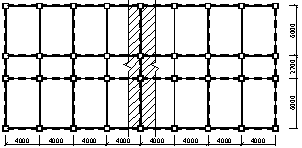(a)    Plane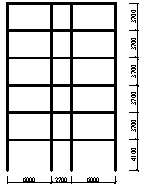(b)    Elevation Figure 2  Structural layout (unit: mm)

The reinforced concrete frame structure shown in Figure 2 is selected to illustrate the collapse risk assessment process. The six-story building is 22.6 m in height, with a seismic fortification category of C, and it is located on a site with a seismic fortification degree of seven (for which the corresponding peak ground acceleration, PGA, corresponding to a 10% exceedance probability in 50 years, is 0.10 g) and a site classification of II . The structure is designed in accordance with the Chinese Code for Seismic Design of Buildings (GB50010-2010)  . A detailed description of its seismic design can be found in the study by Shi et al.  .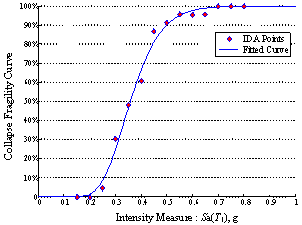Figure 3  Collapse fragility curve of the RC frame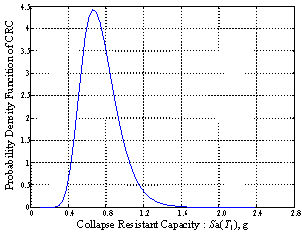Figure 4  Probability density function of CRC

4.2     Collapse fragility analysis

Nonlinear finite element modeling and IDA are performed using TECS (Tsinghua Earthquake Collapse Simulation) [14-18], a program developed based on the general-purpose finite element software MSC.MARC for collapse simulation of complex structures. The collapse fragility analysis adopts the 22 far-field ground motion records proposed in the FEMA 695 report  . The spectral acceleration at the fundamental period Sa(T1) is selected as the intensity measure  . The collapse criterion is defined as the structure losing its vertical bearing capacity such that it is unable to maintain enough space for life safety   .

The serial collapse probabilities at discrete intensity levels are obtained via IDA and are denoted as ��IDA points,�� as shown in Figure 3. If the collapse fragility curve shown in Figure 3 is assumed to follow a lognormal distribution, the characteristic values of the probability distribution can be estimated based on the IDA results, i.e. , m = -1.0257 and s = 0.2596, where m  is the mean value of ln(CRC) and s  is the standard deviation of ln(CRC). Therefore, the cumulative probability distribution function (Figure 3) and the probability density function of CRC (Figure 4) are derived via the following equations, respectively  .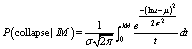(3)(4)

4.3     Seismic hazard analysis

Three locations, Puxian City in Shanxi Province, Rizhao City in Shandong Province and Mojiang City in Yunan Province, are selected to compare the risks of earthquake-induced collapse of the structure described above in different regions. The three regions have the same seismic fortification degree of seven, but their actual seismic hazards are different. The ground motion PGA values corresponding to the serviceable-level earthquake, fortification-level earthquake and collapse-prevention-level earthquake for the three regions, according to China��s seismic hazard characteristic zonation  , are listed in Table 1. The three intensity levels are defined as the exceedance probabilities of 63% in 50 years, 10% in 50 years and 5% in 50 years, respectively. Table 1 shows that the three regions have the same PGA values for the fortification-level earthquake but different PGA values for the serviceable-level and collapse-prevention-level earthquakes.

Because the spectral acceleration at the structural fundamental period Sa(T1) is used as the ground motion intensity measure in this analysis, the PGA values in Table 1 should be converted to corresponding Sa(T1) values so that the results of the collapse fragility analysis can be used to predict the collapse risks. Based on the design response spectrum provided in the Chinese Code for Seismic Design of Buildings  , this transformation is performed as follows: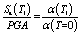(5)

where a(T1) is the value of the seismic influence coefficient corresponding to the structural fundamental period T1 and a(T=0) is the value of the seismic influence coefficient corresponding to the rigid single-degree-of-freedom (SDOF) system, which theoretically makes  a(T=0) equal to PGA/g. The fundamental period T1 of the investigated RC frame structure equals 0.9671 s. The Sa(T1) values derived are also shown in Table 1.

Table 1  Comparison of seismic hazards and collapse probabilities

 Seismic Region Puxian City in Shanxi Province Rizhao City in Shandong Province Mojiang City in Yunnan Province Seismic Fortification Intensity 7 7 7 Character Period Zonation 2 2 2 Hazard Characteristic Zonation I II III Intensity Levels Serviceable PGA/g 0.0145 0.0342 0.0603 Sa(T1)/g 1.456��10-02 3.434��10-02 6.054��10-01 Fortification PGA/g 0.1 0.1 0.1 Sa(T1)/g 1.004��10-01 1.004��10-01 1.004��10-01 Collapse Prevention PGA/g 0.1619 0.1344 0.1163 Sa(T1)/g 1.625��10-01 1.349��10-01 1.168��10-01 Total Collapse Probability in 50 years 2.75% 1.12% 0.14%

The exceedance probability in 50 years represents the possibility that an earthquake beyond the given intensity measure of IM is experienced at the building site at least once in 50 years. Consequently, the seismic hazard curve (i.e., the exceedance probability in 50 years versus IM, denoted as E(IM))) has to satisfy the following two underlying boundary conditions: (a) At the boundary of Sa(T1)=0, the corresponding value E(Sa(T1)=0) should equal 100% because it is almost certain that the building site will be affected by at least one earthquake beyond the intensity level of Sa(T1)=0; and (b) at the boundary of Sa(T1)= +��, the corresponding value E(Sa(T1)= +��) should equal 0% because it is impossible that an earthquake beyond the intensity level of Sa(T1)= +�� is experienced at the building site. To satisfy these boundary conditions and also to make the fitted curve approach the Sa(T1) values listed in Table 1, the seismic hazard curve is fitted using Equation (6). The values of the parameters in Equation (6) are determined by the following two steps: (a) fitting the Sa(T1) values listed in Table 1 with the equation E(Sa(T1))= aSa(T1)b  to obtain the values of parameters a and b; and (b) solving the equation aSa(T1)b=1.0 to find the breaking point Sa(T1)Critical.

 E(Sa(T1)) =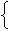1.0, Sa(T1)Sa(T1)Critical

The derived seismic hazard curves are shown in Figure 5. The seismic hazard curves of the three regions intersect at the fortification-level intensity because they have the same seismic fortification intensity of seven. However, the values of E(Sa(T1)) differ significantly at intensity levels other than the fortification-level intensity. Of the three locations, Puxian City in Shanxi Province has the highest probability of experiencing a mega-earthquake which is more intensive than the collapse-prevention-level, while Mojiang City in Yunnan Province has the lowest probability. Because the collapse of the buildings is mainly induced by mega-earthquakes that are stronger than the collapse-prevention-level earthquake, differences in the mega-earthquake hazards for different locations should definitely be considered in design.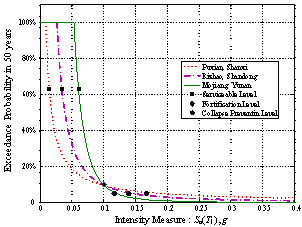Figure 5  Exceedance probability in 50 years versus Sa(T1)

4.4     Comparison of the collapse risks

The risks of earthquake-induced collapse of identical RC frame structures located in the three selected regions with different seismic hazards are evaluated using Equation (2) based on both the probability density function of CRC (Figure 4) and the seismic hazard (Figure 5). The values of the total probabilities of collapse in 50 years are listed in the last row of Table 1.

The three regions have the same seismic fortification degree of seven, and therefore, according to the Chinese Code for Seismic Design of Buildings  , the design requirements for buildings located in the three regions are also the same. This means that the buildings have the same resistance to earthquake-induced collapse. However, because of the different seismic hazards in the three regions, the actual collapse risks of the buildings during their design service lives are quite different.

The intensity measure corresponding to the peak value of the probability density function of CRC as shown in Figure 4 is generally approximately 1 to 2 times larger than the intensity measures of collapse-prevention-level earthquakes (1.06 times larger for Puxian City, 1.48 times larger for Rizhao City and 1.87 times larger for Mojiang City), which means that the collapse of the buildings is mainly induced by mega-earthquakes stronger than collapse-prevention-level earthquakes. The risk of collapse is very small when buildings are subjected to ground motions smaller than a collapse-prevention-level earthquake. Of the three regions discussed above, Puxian City has the smallest serviceable-level earthquake intensity but the largest collapse-prevention-level intensity. Thus, Puxian City has the largest total probability of collapse in 50 years. Mojiang City, in contrast, has the largest intensity for a serviceable-level earthquake and the smallest intensity for a collapse-prevention-level earthquake; its total collapse probability is merely 0.14%.

5.       Suggestions for future seismic design

Evaluation of structural safety against earthquake-induced collapse should consider both collapse fragility analysis and seismic hazard analysis results, which represent the collapse resistant capacity of a structure and the earthquake ground motion demand, respectively. The total collapse probability of a building during its design service life is proposed as the index to evaluate the earthquake collapse risk. The process for assessing the total probability of collapse is described and demonstrated in this paper. With the current Chinese seismic design code, the collapse risk differs greatly from site to site due to variation in the seismic hazard. The full range of seismic hazards, especially including mega-earthquakes, should be considered in seismic design.

The United States has already begun to consider the influence of actual seismic hazards on collapse safety in the updated version of its seismic design code. Luco et al.  proposed to modify the uniform-hazard portions of the seismic design maps in accordance with NEHRP (National Earthquake Hazards Reduction Program) Provisions to achieve a uniform estimated collapse probability. The experience of the United States in this regard is a helpful model for improvement of the Chinese seismic design method.

The objective of uniform-risk-targeted seismic design for collapse safety of building structures is to ensure that building structures have the same collapse risk over a prescribed period no matter where they are located. The collapse risk should be acceptable by the society and the economy. Because the collapse risk evaluation method proposed in this paper is not sufficiently developed for current use in seismic design practice, the following research strategy for developing and implementing uniform-risk-targeted seismic design for collapse safety is recommended.

(a) Seismic design zonation is mapped directly according to the collapse-prevention-level earthquake (e.g., 2% exceedance probability in 50 years), which is based on the results of seismic hazard analysis. Ground motion values corresponding to the collapse-prevention-level earthquake should be used for seismic design. Because the collapse risk is mainly dominated by the exceedance probability of mega-earthquakes (defined as earthquakes beyond the collapse-prevention-level), regions with similar collapse-prevention-level earthquakes should be further subdivided according to the hazard of mega-earthquakes (which can also be referred to as a seismic hazard classification).

(b) The relationship between ground motion values of collapse-prevention-level earthquakes and the corresponding structural load that is used in seismic design should be studied to enable the development of a seismic design for reliably ensuring the collapse safety of buildings subjected to collapse-prevention-level earthquakes. In other words, the design seismic load should be determined based on the collapse-prevention-level earthquake zonation map.

(c) The China��s current seismic design method as well as earthquake-resistant construction measures should be further quantitatively evaluated and improved via collapse fragility analysis according to the seismic hazard classification and seismic design load described in the previous two steps, until the uniform collapse probability is achieved.

6.       Conclusions

The concept and the assessment process for uniform-risk-targeted seismic design are presented in this paper. The collapse risks of example buildings located in different regions with the same fortification level but different seismic hazards are analyzed. The results show that with China��s current seismic design method, the risk of collapse during a building��s design service life may differ greatly among different regions. Additional research is required to develop the uniform-risk-targeted seismic design approach proposed in this paper.

Acknowledgement

This work was supported by the National Natural Science Foundation of China (Grant Nos. 90815025, 51178249), the Tsinghua University Research Funds (Grant Nos. 2010THZ02-1, 2010Z01001) and the Program for New Century Excellent Talents in University (Grant No. NCET-10-0528).

Appendix

The following derivation is provided to prove the equivalence of the following equations.(1)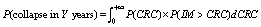(2)

To facilitate the mathematical expressions of Equations (1) and (2), the probabilistic functions are expressed as(7)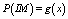(8)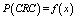(9)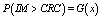(10)

P(collapse|IM)=F(x) is the conditional collapse probability at given values of the intensity level, which equals the cumulative distribution function of CRC as discussed in the paper. P(CRC)=f(x) can be treated as the probability that the structure��s collapse resistant capacity equals CRC, which is also the probability density function of CRC. Therefore, F(x) and f(x) should satisfy the following equation.(11)

P(IM)=g(x) is the probability density function that the building site experiences earthquakes of intensities equal to IM during the design service life. P(IM>CRC)=G(x) is the likelihood that the building site experiences earthquakes with intensities larger than CRC during the design service life. Thus, g(x) and G(x) satisfy the following equation.(12)

After integrating Equation (1) by parts and inserting Equation (12) into it, the following equation is obtained.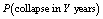(13)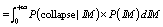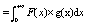Forin Equation (13), when x=+��, F(x)=P(collapse|IM) can be regarded as the conditional collapse probability when a building is subjected to an earthquake with IM=+�� and thus it equals one. G(x)=P(IM>CRC) is the likelihood that the building site experiences earthquakes with IM greater than +�� and thus G(x)=P(IM>CRC) equals zero. Similarly, when x=0, F(x)=P(collapse|IM) can be regarded as the conditional collapse probability when a building is subjected to an earthquake with IM=0 and thus F(x)=P(collapse|IM) equals zero. G(x)=P(IM>CRC) is the likelihood that the building site experiences earthquakes with IM larger than 0 and thus equals one. Therefore,(14)

Substituting Equations (11) and (14) into Equation (13) leads to the following equation.(15)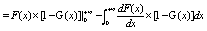Because f(x)=P(CRC) denotes the probability density function of the collapse resistant capacity,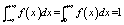(16)

Substituting Equation (16) into Equation (15) yields(17)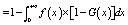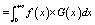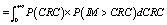The equivalence of Equation (1) and Equation (2) has been proven.

References

      Zareian F, Krawinkler H. Assessment of probability of collapse and design for collapse safety. Earthquake Engineering and Structural Dynamics, 2007, 36(13): 1901�C1914.

      Vamvatsikos D, Cornell C A. Incremental dynamic analysis. Earthquake Engineering and Structural Dynamics, 2002, 31(3): 491-514.

      Nicolas L, Ellingwood B R, Ronald O H, etc. Risk-targeted versus current seismic design maps for the conterminous United States. SEAOC 2007 Convention Proceedings.

   Gao M T, Lu S D. The Discussion on principles of seismic zonation of the next generation. Technology for Earthquake Disaster Prevention, 2006, 1(1): 1-6. (in Chinese)

   McGuire R K. Probabilistic seismic hazard analysis and design earthquakes: closing the loop. Bulletin of the Seismological Society of America, 1995, 85(5): 1275-1284.